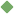quantum 1. [ noun ] how much there is of something that you can measure Synonyms: amount quantity measure Related terms: abstraction time_period probability volume value time_interval point fundamental_measure volume proof time_unit system_of_measurement playing_period octane_number cordage relative_quantity definite_quantity magnetic_flux linear_measure indefinite_quantity magnetisation measure total standard nonstandard To share this definition press "text" (Facebook, Twitter) or "link" (blog, mail) then paste text link 2. [ noun ] (mathematics) a discrete amount of something that is analogous to the quantum in quantum theory Related terms: quantity 3. [ noun ] (physics) the smallest quantity of some physical property that a system can possess (according to quantum theory) Related terms: measure quantity quasiparticle physics Similar spelling: quondam   quantong